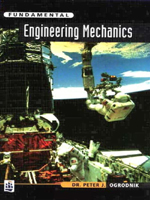Fundamental Engineering Mechanics

By Peter Ogrodnik
March 1997
Pearson Education
ISBN: 0582297990256 pages, Illustrated
\$72.50 Hardcover

OUT OF PRINT

This unique book provides a basic practical introduction to engineering mechanics and is written specifically for those students who need a thorough grounding in the subject to participate fully in their engineering course. The book satisfies the needs of many foundation year engineering courses as well as many other engineering courses.

Contents
1. Units. 2. Velocity and acceleration. 3. Constant acceleration. 4. Newton's laws, momentum and force. 5. Work, energy and power. 6. Modeling using free-body diagrams. 7. Thermo-fluid applications. 8. Structural analysis. 9. Behavior of materials. 10. Case studies. Appendices. A: Standard trigonometric functions. B: Standard differentials and integrals. C: Numerical differentiation and integration. D: Gravitational acceleration. E: Questions from past exam papers.

Features " Introduces fundamental engineering principles and relates them to real-life examples. " Includes questions (with answers) at end of each chapter. " Takes step-by-step approach to problem solution. " Maths presented as engineering tools rather than subjects in their own right. " Excel spreadsheets available to lecturers adopting the text. " Complements Hannah & Hillier's Applied Mechanics, and links with Croft & Davison's Foundation Maths.